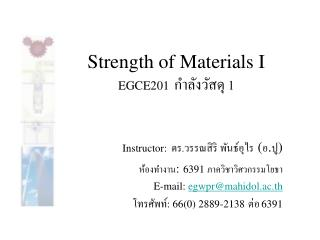DownloadDownload PresentationStrength of Materials I EGCE201 กำลังวัสดุ 1

# Strength of Materials I EGCE201 กำลังวัสดุ 1

Download Presentation## Strength of Materials I EGCE201 กำลังวัสดุ 1

- - - - - - - - - - - - - - - - - - - - - - - - - - - E N D - - - - - - - - - - - - - - - - - - - - - - - - - - -
##### Presentation Transcript

1. Strength of Materials I EGCE201กำลังวัสดุ 1 Instructor: ดร.วรรณสิริ พันธ์อุไร (อ.ปู) ห้องทำงาน:6391 ภาควิชาวิศวกรรมโยธา E-mail: egwpr@mahidol.ac.th โทรศัพท์: 66(0) 2889-2138ต่อ6391

2. Symmetric Bending of Beams • A beam is any long structural member on which loads act perpendicular to the longitudinal axis. Learning objectives • Understand the theory, its limitation and its applications for strength based design and analysis of symmetric bending of beams. • Develop the discipline to visualize the normal and shear stresses in symmetric bending of beams.

3. Pure Bending Independent of material model

4. Deformation of symmetric member Under action of M and M’, the member will bend but will remain symmetric with respect to the plane containing the couples. except

5. compressed (compression) elongated (tension) There exists a surface // to the upper and lower faces of the member (see as a line on the cross-section) where no elongation and the bending normal stress is zero. This surface is called the neutral axis.

6. The length of arc DE for both deformed and undeformed L = rq Consider an arc some distance y above the neutral surface The length of arc JK can be expressed as L’ = (r- y)q

7. The deformation d of JK d = L’-L = (r- y)q - rq = - yq The normal strain is max when y is the largest

8. Derive flexure formula Because From earlier,

9. Derive flexure formula (continued) This equation can be satisfied only if The first area moment of the cross section about its NA = 0

10. Derive flexure formula (continued) Taking the moment about the z axis = 0 is the 2nd area moment of the cross section w.r.t. z axis

11. Discuss flexure stress Compression Top Surface (+y) Tension Bottom Surface (-y)

12. Not only bending about z axis produces a normal stress in x direction but also bending about y axis. If the bending moment is about the y axis, a similar relationship exists.

13. Example

14. Compute the area moment of inertia

15. A normal stress in the x direction due to Mz

16. A normal stress in the x direction due to My

17. Transverse Deformations due to bending

18. Member of several materials • Assume a bar is made of two different materials bonded together. The bar will deform as previously shown. • The normal strain in x direction will vary linearly with distance from the N.A.

19. Method of transformed section The resistance to bending would be the same if each section were made of the same material,where the 2nd material was multiplied by n

20. Example – transformed section to all aluminum

21. Season’s Greeting! Try it for yourself at home Transform section to all steel

22. Beams bending analysis • Beams carry loads perpendicular to their longitudinal axis. • Internal shear forces and • bending moments develop • along the span of a beam. In designing a beam, it is critical to determine the internal shear force (V) and bending moment distribution (M). This is accomplished by constructing shear and bending moment diagrams.

23. Steps in constructing a V and M diagram In general, the load distribution across the width of the beam is assumed to be applied uniformly. Therefore, a beam can be analyzed in 2 dimensions rather than 3. 1. Determine the reactions at each support.

24. Review of support conditions

25. Shear forces and bending moments in beams Neither half is in equilibrium

26. The force imbalance that exists must be counteracted so that static equilibrium is maintained. This is done Through internal forces and moments.

27. Calculating internal V and M as a function of x by isolating a segment of beam a distance x from the left end whose width is dx.

28. = eliminate last term = 0

29. Diagram by inspection V = constant M = f(x) V = f(x) M = f(x2) V = no effect M = spike

30. Faculty of Engineering Mahidol University, Thailand Dr. Wonsiri Punurai (Bo)